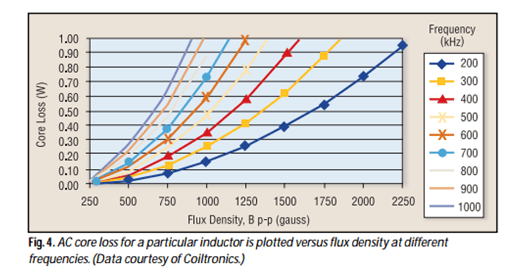# Power Losses in Boost Converter

### Power losses in boost converter are due to the following contributors:

1. Conduction loss of MOSFET
2. Turn on and turn off losses of MOSFET
3. Gate charge or input capacitance loss
4. Output capacitance loss
5. Diode loss
6. Inductor loss
7. Bias losses

For AC-DC application, rectifier loss will be taken into account as well.

## Detailed Explanation on Power Losses in Boost Converter

### Conduction Loss of MOSFET

This is due to the channel on state resistance or commonly known as the RDSon. RDSon is dependent to the applied gate-source voltage (VGS) and the junction temperature.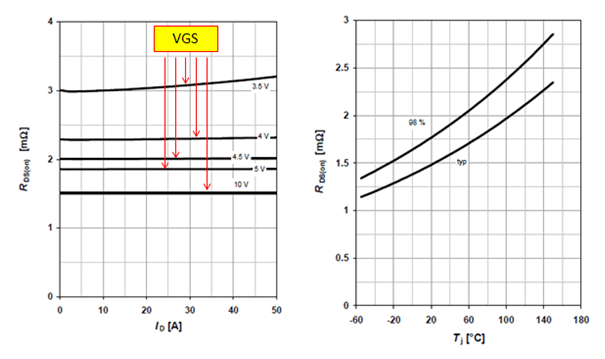The power loss equation used to compute RDSon loss is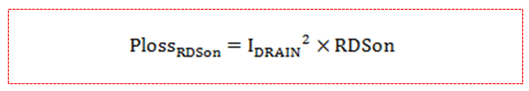Where IDRAIN is the RMS drain current while RDSon is the right on-state resistance considering VGS and the junction temperature.

Considering below boost circuit, the RMS drain current is can be computed using below equation: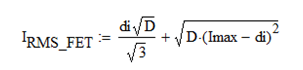WhereWhere;

Iout – DC load current of the boost converter

Fsw – Switching frequency

L1 – boost inductor

Vin – input voltage

VD – forward drop of the diode

VS – voltage drop of the MOSFET

Vout – output voltage

### Turn On and Turn Off Losses of MOSFET

This is associated to the rise and fall times of the MOSFET drain voltage.The power loss is approximated using below equation:Where;

trise – rise time

tfall – fall time

IRMS_FET – RMS current of the FET

VDS_FET – drain voltage of the FET

Fsw –switching frequency

### Gate charge or input capacitance loss

Gate charge loss is dictated by the input capacitance of the FET. Datasheet may provide input capacitance or the total gate charge. I prefer to use the total gate charge.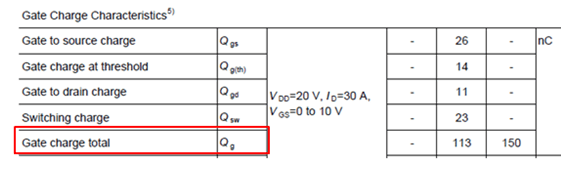The power loss is can be computed using the equation below.Where;

Qgtotal – total gate charge

VGS – applied gate to source voltage

Fsw – switching frequency

### Output Capacitance Loss

This is related to the COSS of the MOSFET. This parasitic capacitance is dependent on the drain voltage. For worst case, the maximum value is can be considered. For efficiency calculation however, the right value must be used to get a realistic result.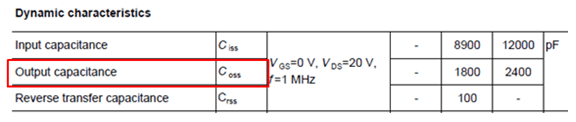Power loss due to COSS is can be calculated using below equation: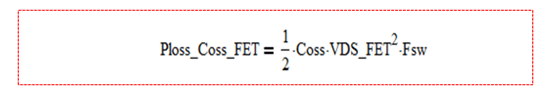Where;

Coss – output capacitance of the FET

VDS_FET – drain voltage of the FET

Fsw – switching frequency

### Diode Loss

For the diode, the loss due to forward voltage is given by the equation below:Where IRMSDIODE is the RMS current through the diode while VF is the forward voltage of the diode

Considering below circuit, the RMS current of the diode is given by the equation below.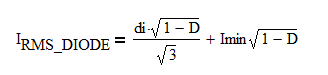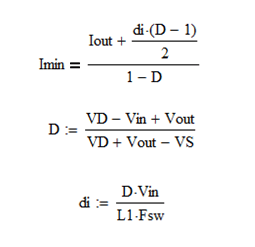Where;

Iout – DC load current of the boost converter

Fsw – Switching frequency

L1 – boost inductor

Vin – input voltage

VD – forward drop of the diode

VS – voltage drop of the MOSFET

Vout – output voltage

Reverse recovery on the diode also contribute a small loss. It is important to select a very fast switching diode.

### Inductor loss

There are two contributors of losses in an inductor. One is the wire loss and the second is the core loss. The former is a DC loss while the latter is an AC loss.

The loss due to the wire is given by the equationConsidering below circuit, the RMS current of the inductor is given below.whereWhere;

Iout – DC load current of the boost converter

Fsw – Switching frequency

L1 – boost inductor

Vin – input voltage

VD – forward drop of the diode

VS – voltage drop of the MOSFET

Vout – output voltage

The losses due to the core is straight forward for an off the shelf inductor. There is a graph between flux density and core loss in different frequency the same as below. For instance at 200kHz switching frequency, at a flux density of 1500 gauss, the core loss is around 0.4W.For very high power application, core loss represent the significant portion of the total power losses in boost converter.

### Bias Losses

Bias losses are the fix losses on the divider resistors, signal diodes, driver circuits, protection circuits, monitoring or housekeeping circuits and the likes. These losses however are very small compared to above losses.

The total power losses in boost converter are the sum of all factors enumerated above. For lower frequency operation, conduction loss of the MOSFET and forward loss of the diode prevails. For high frequency operation, the switching and AC losses dominate. The system efficiency is can be computed using the equation1. Ann says:

@author: JLSORONIO
Can you share your source of information? Where did you deduce the formulas above?

Cheers!

1. jlsoronio says:

Ann,

As a power electronics engineer, I consider reading different app notes and books. However, what I got are only principles and basic equations. I want to know myself the equations to be used to calculate the losses of any switching converters. Because of this goal, I did my own analysis and derivations…In short, I derived the equations of the currents and duty cycle. I proven that equations correct through simulations.

2. Chetan says:

Just curious , what is the reason for your cross questioning. dont you trust these eq. i didnt check all but from engineering prospective switching loss for boost converter are good.

This site uses Akismet to reduce spam. Learn how your comment data is processed.